University of Wisconsin – Madison

# Chemical Production Scheduling

In chemical production scheduling, we seek to allocate limited resources to competing tasks over time. The key decisions include the selection and sizing of tasks (batches), the assignment of tasks to equipment units, and the timing of tasks. Production planning and scheduling problems arise in many areas, from basic chemicals and consumer products to pharmaceuticals and specialty chemicals.

We classify chemical production scheduling problems by introducing triplet ?/?/?, where

? denotes the production environment. Chemical production environments are classified into three categories, namely network, sequential, and hybrid. Sequential environment can be further classified into multistage and multipurpose plants.

? denotes the processing restrictions and characteristics, such as setups, changeovers, storage constraints, material transfers. Note that multiple restrictions and characteristics can be present simultaneously in a problem.

? denotes the objective function, including both minimization (makespan, cost, tardiness, etc.) and maximization (profit, production, etc.) objectives.

The goal of our research is to address problems of industrial size by formulating mixed-integer programming (MIP) models that can address the limitations of existing models and developing advanced solution strategies. Furthermore, we aim to understand the characteristics of online scheduling, where the schedule is constantly updated by repeatedly solving the problem, whenever a trigger event occurs or as the scheduling horizon advances. Specifically, we are investigating how different attributes of each open loop schedule affect the overall quality of the closed loop solution.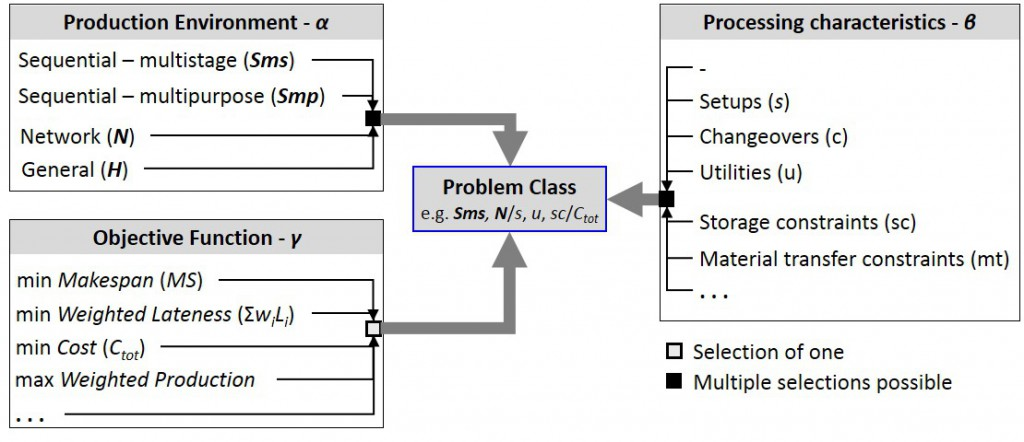Figure 1. Problem classes in chemical production scheduling. Partial list of processing characteristics and restrictions (?), and objective functions (?) are shown.

Generally, chemical production environments can be broadly classified into three categories, namely network, sequential, and the combination of the two, i.e. hybrid. Different material handling restrictions are present in each production environment, leading to different modeling approaches used for scheduling in these environments.

1) Sequential environment: Due to strict material handling restrictions (no-mixing/splitting of batches) mainly batch-based modeling approach is adopted. Unlike the majority of the models developed, we study MIP models for both multistage and multipurpose plants that can simultaneously account for batching and scheduling decisions, thereby leading to better solutions (Sundaramoorthy et al., 2009). The discrete-time representation that we adopt in the models offer many advantages such as linear modeling of inventory and resource utilization profiles, straightforward modeling of time-varying resource availability and cost, etc (Merchan et al., 2016).

2) Network environment: Batches of material can be freely mixed and split, thereby material-based modeling approach is adopted in this environment. We study new continuous-time representations that do not require tasks to start or end at a time point, reducing the number of time points needed for a solution. In addition, we introduce inventory variables for processing units, which allows us to model non-simultaneous and partial material transfers (Gimenez et al., 2009).

3) Hybrid environment: In hybrid environment, each material have different material handling restriction. Thus, both aspects of network and sequential environments are present in the system. While previous studies have solely focused on either sequential or network environment, we develop an approach that can handle hybrid processes by introducing different type of material balance and handling constraints (Sundaramoorthy and Maravelias, 2011; Velez and Maravelias, 2013). An example of a problem that can now be addressed using the new formulation is given in Figure 1.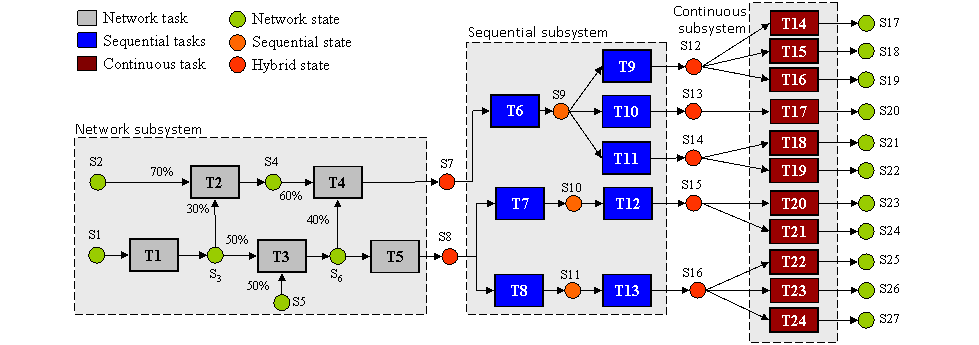Figure 1. Complex chemical facility consisting of different subsystems

Reference:

Gimenez, D. M., Henning, G. P. and Maravelias, C. T. (2009) A novel network-based continuous-time representation for process scheduling: Part I. Main concepts and mathematical formulation. Computers & Chemical Engineering. 33, 1511-1528.

Merchan, A. F., Lee, H. and Maravelias, C. T. (2016) Discrete-Time Mixed-Integer Programming Models and Solution Methods for Production Scheduling in Multistage Facilities. Computers and Chemical Engineering.

Sundaramoorthy, A. and Maravelias, C. T. (2011) A General Framework for Process Scheduling. Aiche Journal. 57, 695-710.

Sundaramoorthy, A., Maravelias, C. T. and Prasad, P. (2009) Scheduling of Multistage Batch Processes under Utility Constraints. Industrial & Engineering Chemistry Research. 48, 6050-6058.

Velez, S. and Maravelias, C. T. (2013) Mixed-Integer Programming Model and Tightening Methods for Scheduling in General Chemical Production Environments. Industrial & Engineering Chemistry Research. 52, 3407-3423.

Despite advances in computer hardware and optimization software, the scheduling of chemical processes remains a hard problem. One of the goals of our research is to formulate mixed-integer programming (MIP) models for a wide range of scheduling problems. Since the solution of MIP scheduling models is computationally challenging, we aim to develop advanced solution methods:

1)  Tightening methods: Based on customer demand, process network characteristics and time related data, we calculate the total amount of material and number of batches each task must produce, as well as the number of times subsets of tasks can be executed in a feasible solution. Based on these amounts, we generate tightening constraints (Velez et al., 2013a; Merchan and Maravelias, 2016).

2)  Multi-grid discrete-time models: We formulate models based on separate grids for each task, processing unit, material, and utility. The proposed models have fewer binary variables and constraints than models based on a single and uniform time grid, thereby leading to faster solution times (Velez and Maravelias, 2015).

3)  Parallel branch-and-bound: We develop methods where (1) the full MIP is partitioned into many smaller MIPs by fixing or bounding the number of times each task runs, and (2) each smaller MIP is solved in parallel (Velez and Maravelias, 2013b).

4) Reformulations: We study extended reformulations where a new integer variable representing the total number of batches for each task is introduced. Branching on this new variable prevents the search from looking at many equivalent solutions and improves the bound on the optimal solution quickly (Velez and Maravelias, 2013c; Merchan et al., 2016).

5) Solution refinement method: We study a method that enable us to improve the quality of the solutions obtained from discrete-time formulations. We refine the discrete-time solution by re-optimizing the timing of events using a continuous-time model, while keeping the integer decisions fixed. Thus, hard instances can be solved faster with large discretization steps without any loss in accuracy (Merchan et al., 2016).

These methods can improve solution times by several orders of magnitude thereby allowing us to solve in minutes large-scale problems that were intractable (see Figures 1, 2 and 3).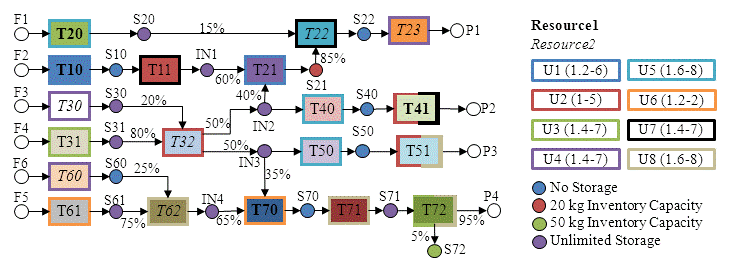Figure 1. Example process network. ?ask outline color indicates which units can process it; font style indicates which resources are required. The color of each material indicates storage policy; unit capacities are given in parentheses.Figure 2. Solution to the instance shown in figure 1. A. Resource profile: total amount of each resource available, total amount in use, and cost. B. Inventory profile; feeds are received every 24 hours for the first 72 hours, and products have due dates every 24 hours starting at 48 hours. C. Gantt chart; tasks in the STN representation have the same color as the corresponding block in the Gantt chart.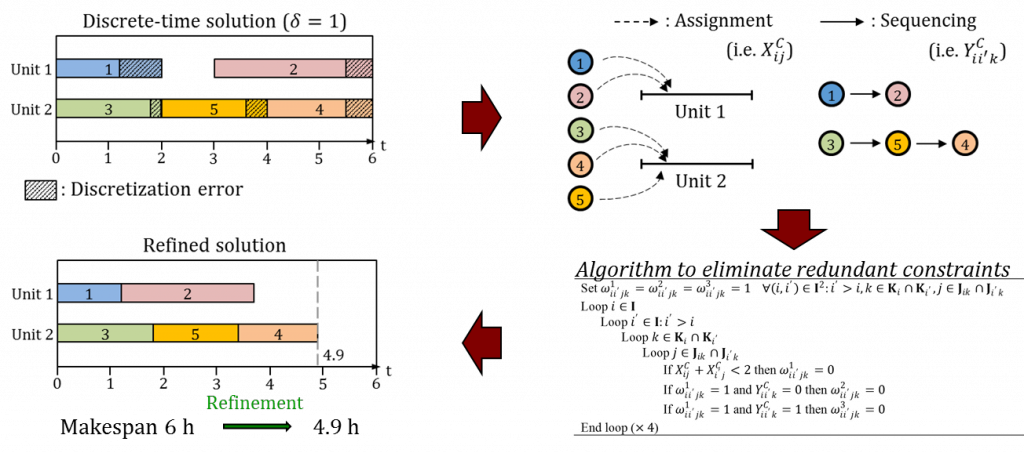Figure 3. An illustration of solution refinement method. The objective value of discrete-time solution obtained using large discretization step of 1 hour is improved from makespan of 6 hours to 4.9 hours after the refinement, which proved to be the optimal solution when fine discretization of 0.1 hour is used.

Reference:

Merchan, A. F., Lee, H. and Maravelias, C. T. (2016) Discrete-Time Mixed-Integer Programming Models and Solution Methods for Production Scheduling in Multistage Facilities. Computers and Chemical Engineering.

Merchan, A. F. and Maravelias, C. T. (2016) Preprocessing and tightening methods for time-indexed MIP chemical production scheduling models. Computers & Chemical Engineering. 84, 516-535.

Velez, S., Sundaramoorthy, A. and Maravelias, C. T. (2013a) Valid Inequalities Based on Demand Propagation for Chemical Production Scheduling MIP Models. Aiche Journal. 59, 872-887.

Velez, S. and Maravelias, C. T. (2013b) A branch-and-bound algorithm for the solution of chemical production scheduling MIP models using parallel computing. Computers & Chemical Engineering. 55, 28-39.

Velez, S. and Maravelias, C. T. (2013c) Reformulations and Branching Methods for Mixed-Integer Programming Chemical Production Scheduling Models. Industrial & Engineering Chemistry Research. 52, 3832-3841.

Velez, S. and Maravelias, C. T. (2015) Theoretical framework for formulating MIP scheduling models with multiple and non-uniform discrete-time grids. Computers & Chemical Engineering. 72, 233-254.

Since solving MIP scheduling models can be computationally expensive, we study the structure of MIP models to derive combinatorial insights for these formulation that would allow us to develop better solution methods. We investigate how chemical manufacturing facilities can be represented as dynamic networks and then converted into time-expanded networks with side constraints (Maravelias, 2012) (see Figure 1).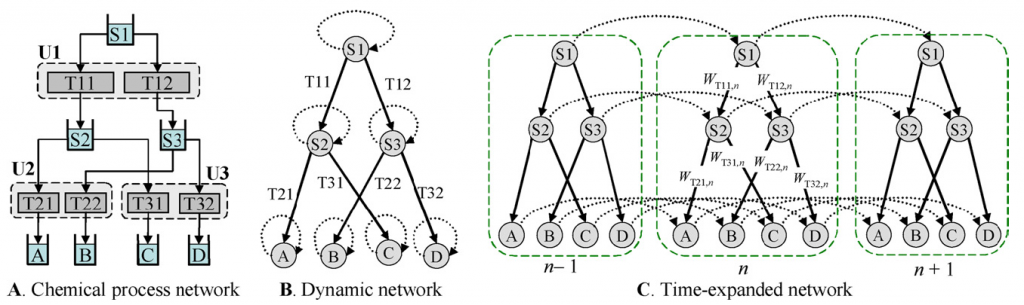Figure 1. Representation of chemical facility as a dynamic network and then time-expanded network. The assignment constraints are written as the side constraints in the time-expanded network.

One of the common characteristics in chemical processes is the presence of sequence-dependent changeover times. Unfortunately, accounting for changeovers leads to the MIP models that are computationally expensive. To address this issue, we propose five new formulations for sequence-dependent changeovers. In terms of theory, we first prove nine propositions regarding the relative tightness of different formulations (see Figure 2). Second, we prove that one of the formulations, (SIIT), is facet-defining for the problem that is subject to changeover and unit availability constraints. In terms of computation, some of the new formulations outperform the formulations in literature. Interestingly, we find that tighter formulations do not always lead to faster solution times (Velez et al., 2017).Figure 2. The relative tightness of sequence-dependent changeover formulations. White indicates that the formulation on the left is tighter than the formulation on the top; gray indicates the opposite. A dotted block indicates that neither formulation is tighter. Each letter(s) represent different changeover formulations: (K)/(KB) – Kondili et al. (1993), (SH) – Shah et al. (1993), (W) – Wolsey (1997), (SI)/(SII)/(SIII)/(SIIT)/(P) – Proposed formulation).

Reference:

Maravelias, C. T. (2012) On the combinatorial structure of discrete-time MIP formulations for chemical production scheduling. Computers & Chemical Engineering. 38, 204-212.

Velez, S., Dong, Y. and Maravelias, C. T. (2017) Changeover Formulations for Discrete-Time Mixed-integer Programming Scheduling Models.

In the last two decades there has been an increasing thrust towards using advanced optimization-based methods to construct better schedules. Finding the schedule once, however, is only part of the whole process. Due to disruptions or arrival of new information, the incumbent schedule can become suboptimal or even infeasible, thus motivating the need for online (re)scheduling. Accordingly, we are investigating how the design of the online scheduling problem (the open-loop problem), and the frequency at which it is solved, affects the quality of the actual implemented schedule (the closed-loop schedule). The ultimate goal is to find the best “online scheduling algorithm”. We have developed a framework for analyzing the relationship between the open-loop problem and the quality of the resulting closed-loop schedule. Through the use of this framework, we have shown, for example, that modifications made keeping an open-loop implementation in mind, do not necessarily result in an improved closed-loop performance. This counter-intuitive observation, along with many other interesting findings, suggest that online scheduling is poorly understood – it is a confounding problem ripe with challenges which, through the efforts in our group, we are now just beginning to understand.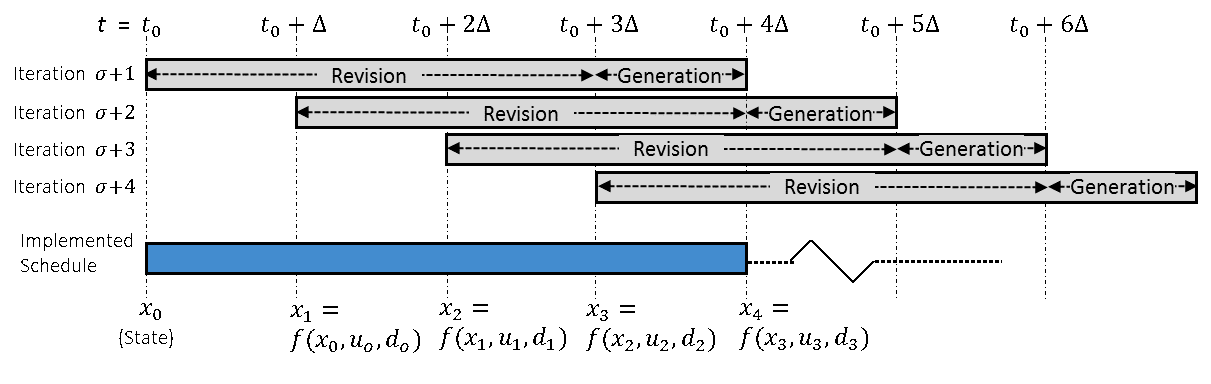Figure 1: Online scheduling using a moving (rolling) horizon. Schedule revision and further schedule generation happen in the same iteration. State of the plant represents complete information of the “current status” as a result from the previous state, the scheduling decisions, and disturbances.